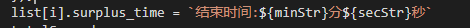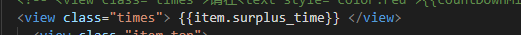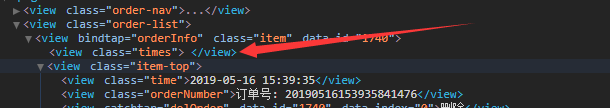# 置顶变量在view输出不了精选热门

大家好这个变量输出不了，有大神知道什么原因吗1 个回答

•undefined
2019-05-16

来个代码片段吧  看也看不出来

2019-05-16
赞同
回复 2
•厚非
2019-05-16
getOrder(status, page) { if (status === -1) status = undefined return new Promise(resolve => { app.api.apiA.orderList({user_id: app.data.user_id, status, page}).then(res => { if (res.response === 'data') { const list = res.list // 格式化时间戳 for (let i = 0; i < list.length; i++) { var totalSecond = list[i].order_end_time - Date.parse(new Date()) / 1000; var interval = setInterval(function () { // 秒数 var second = totalSecond; // 天数位 var day = Math.floor(second / 3600 / 24); var dayStr = day.toString(); if (dayStr.length == 1) dayStr = '0' + dayStr; // 小时位 var hr = Math.floor((second - day * 3600 * 24) / 3600); var hrStr = hr.toString(); if (hrStr.length == 1) hrStr = '0' + hrStr; // 分钟位 var min = Math.floor((second - day * 3600 * 24 - hr * 3600) / 60); var minStr = min.toString(); if (minStr.length == 1) minStr = '0' + minStr; // 秒位 var sec = second - day * 3600 * 24 - hr * 3600 - min * 60; var secStr = sec.toString(); if (secStr.length == 1) secStr = '0' + secStr; this.setData({ countDownDay: dayStr, countDownHour: hrStr, countDownMinute: minStr, countDownSecond: secStr, }); //这里是获取倒计时 list[i].surplus_time = `结束时间:\${minStr}分\${secStr}秒` totalSecond--; if (totalSecond < 0) { clearInterval(interval); wx.showToast({ title: '订单已取消', }); //刷新订单列表 this.orderList(-1) // this.setData({ // countDownDay: '00', // countDownHour: '00', // countDownMinute: '00', // countDownSecond: '00', // }); list[i].surplus_time = `0分0秒` } }.bind(this), 1000); list[i].create_time = app.dateformat(list[i].create_time * 1000) } this.setData({total_page: res.total_page}) console.log(list) resolve(list) } }) }) },
2019-05-16
回复
•拾忆
2019-05-16回复厚非
2019-05-16
回复